SEARCH HOMEMath Central Quandaries & QueriesQuestion from Beth, a student: Hi! (i) Find the number of distinct permutations that can be formed from all of the letters of the word ELEVEN. (ii) How many of them begin and end with E? (iii) How many of them have the 3 E's together? (iv) How many begin with E and end with N? Thanks!!!Hi Beth. I'm going to solve the problem for the word SEVENTEEN and you can do it for ELEVEN.

First, I count up all the letters: I have 9 letters.
Then I look for duplicates: 4 E's and 2 N's.

Now I use the "permutations with duplicates" formula, which says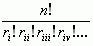where n is the total number of letters and ri, rii, etc are the repeating counts. So in this case I have two repeating letters, so ri = 4 and rii = 2. So the answer to the first part of your question is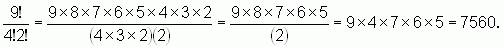For the second part of your question (how many begin and end with an E), I just remove the two E's that have to go at the beginning and the end and I don't think about them anymore. So now I do the same calculation for SVNTEEN: 7 letters with 2 E's and 2 N's: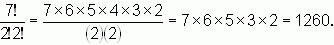For the third part of your question, I just group three of the E's together and think of it as a single "letter". So the "letters" I have are: "EEE",S,V,N,T,E,N: 7 letters with 2 N's. Use the same equation: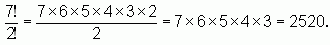And for the last part of the question: "How many begin with E and end with N?", I remove an E and an N from and ignore them because they each have only one place to go. So left over I have SVETEEN: 7 letters, with 3 E's: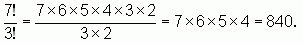Now you try ELEVEN.

Cheers,
Stephen La Rocque.Math Central is supported by the University of Regina and The Pacific Institute for the Mathematical Sciences.